Home > CCA2 > Chapter 8 > Lesson 8.3.2 > Problem8-148

8-148.
1. Given the polynomial p(x) = x3 − 6x2 + 7x + 2. Homework Help ✎

1. What is p(2)?

2. Use the Remainder Theorem to find one factor of x3 − 6x2 + 7x + 2. (See the Math Notes box in Lesson 8.3.2 above.)

3. Use (b) to find another factor.

4. What are all the solutions of x3 − 6x2 + 7x + 2 = 0?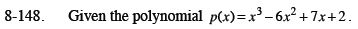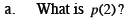Substitute 2 into the equation for every x.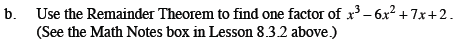Since p(2) = 0, x = 2 is the zero of the function. What is the corresponding factor?

(x − 2)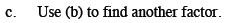Try using a generic rectangle.

(x2 − 4x − 1)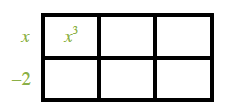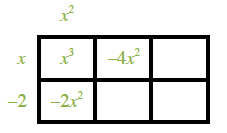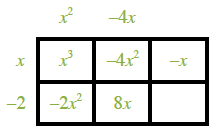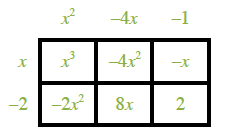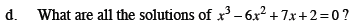See part (a) for one solution.

$x = 2, 2 \pm \sqrt{5}$

Use the Quadratic Formula to find the solutions to 0 = x2 − 4x − 1.

$x=\frac{4 \pm \sqrt{16 + 4}}{2}$